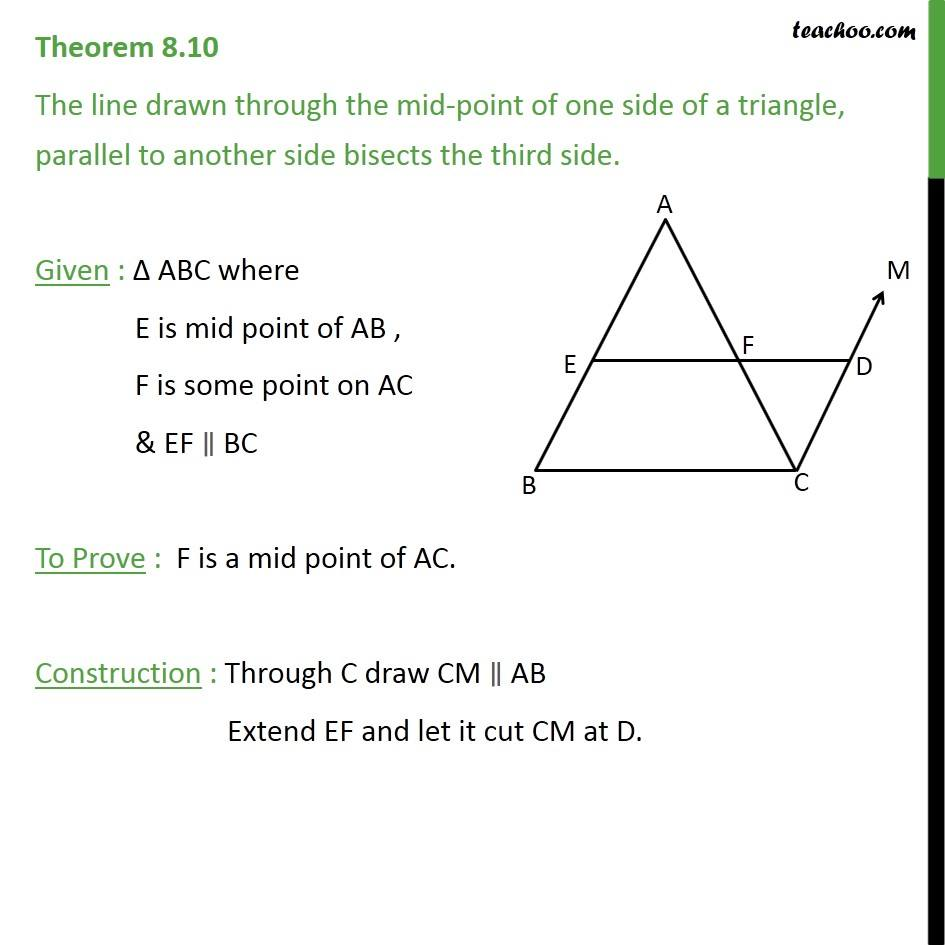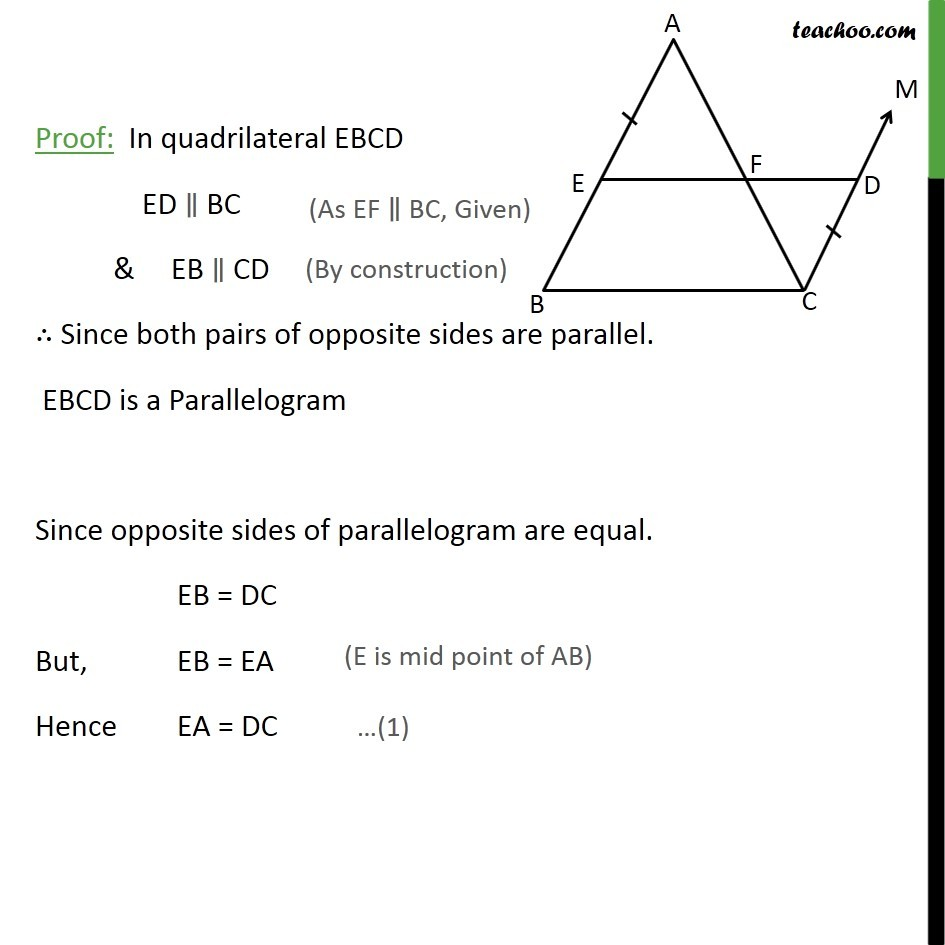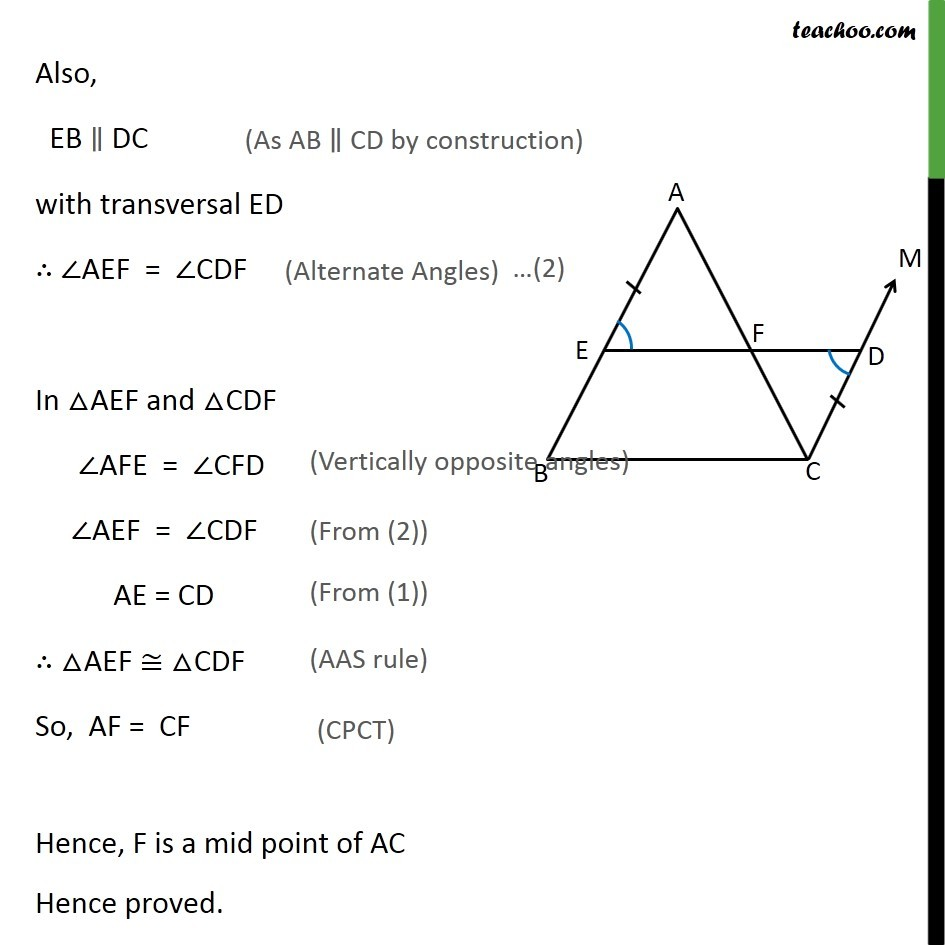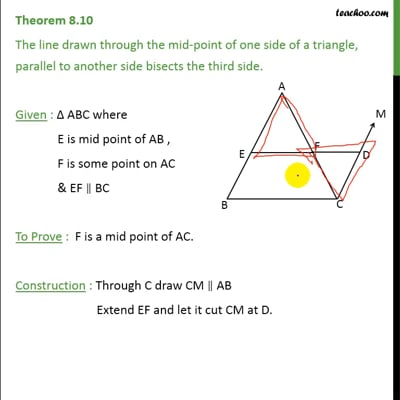Theorems

Serial order wiseThis video is only available for Teachoo black users

Learn in your speed, with individual attention - Teachoo Maths 1-on-1 Class

### Transcript

Theorem 8.10 The line drawn through the mid-point of one side of a triangle, parallel to another side bisects the third side. Given : ABC where E is mid point of AB , F is some point on AC & EF BC To Prove : F is a mid point of AC. Construction : Through C draw CM AB Extend EF and let it cut CM at D. Proof: In quadrilateral EBCD ED BC & EB CD Since both pairs of opposite sides are parallel. EBCD is a Parallelogram Since opposite sides of parallelogram are equal. EB = DC But, EB = EA Hence EA = DC Also, EB DC with transversal ED AEF = CDF In AEF and CDF AEF = CDF AFE = CFD AE = CD AEF CDF So, AF = CD Hence, F is a mid point of AC Hence proved.